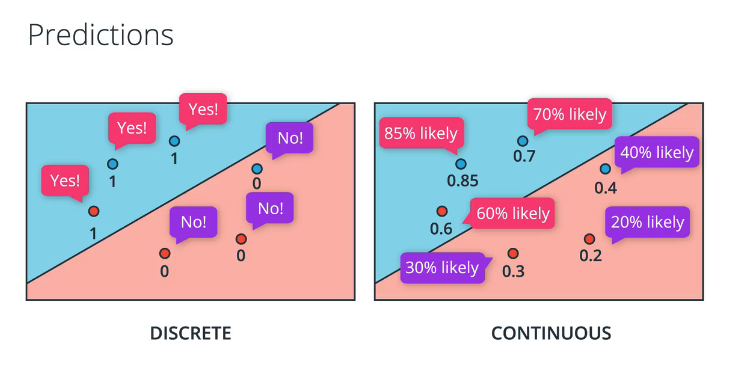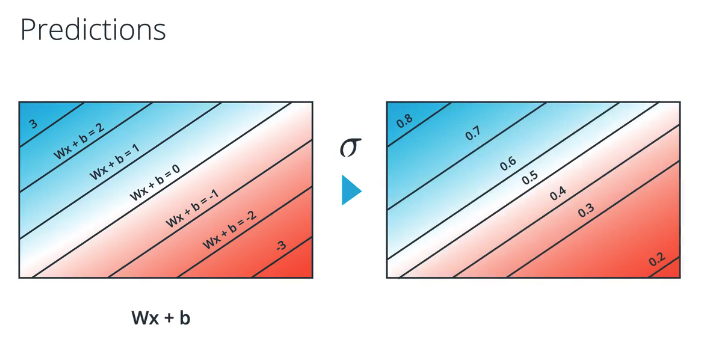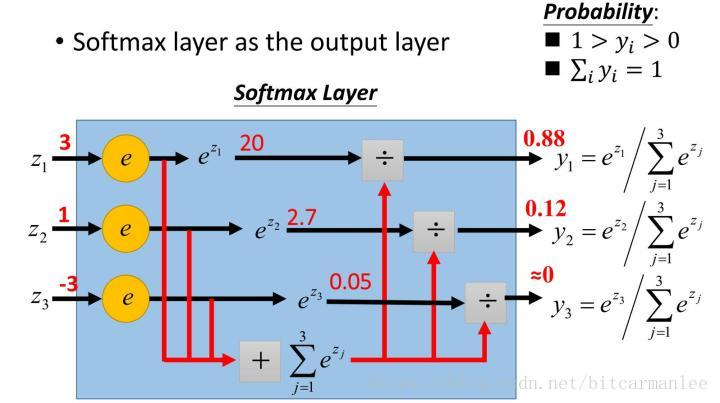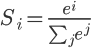# Python:离散型与连续性预测与 softmax 以及 One-Hot 编码 (五十八)

## 离散型与连续型预测

### sigmoid 函数## 多类别分类和 Softmax函数

### 2.softmax的定义### 练习：编写 Softmax

Softmax 公式：import numpy as np

# Write a function that takes as input a list of numbers, and returns
# the list of values given by the softmax function.
def softmax(L):
expL = np.exp(L)
print(expL)
sumExpl = sum(expL)
print("sum:")
print(sumExpl)
print("=====sum:=====")
result = []

for i in expL:
print(i)
result.append(i*1.0 / sumExpl)

return result    
结果打印：
    <type 'numpy.ndarray'>
[ 148.4131591   403.42879349 1096.63315843]
sum:
1648.47511102
=====sum:=====
148.413159103
403.428793493
1096.63315843
Trying for L=[5,6,7].
The correct answer is
[0.09003057317038046, 0.24472847105479764, 0.6652409557748219]
And your code returned
[0.09003057317038046, 0.24472847105479764, 0.6652409557748219]

Correct!

## One-Hot编码

### One-hot Encoding